Question

stuck on part b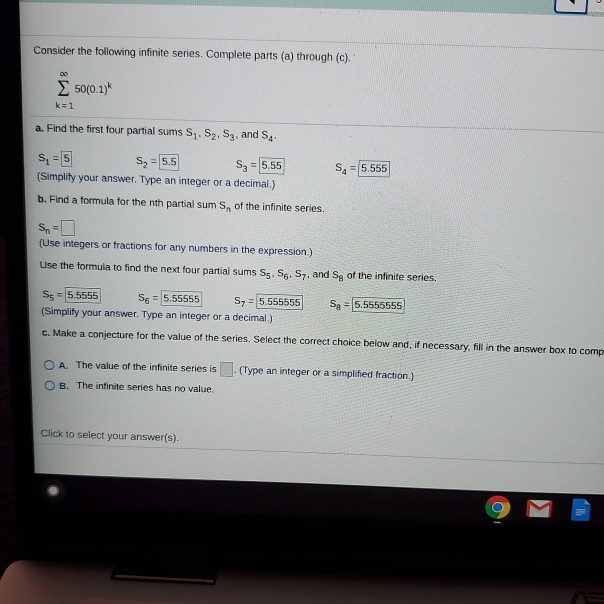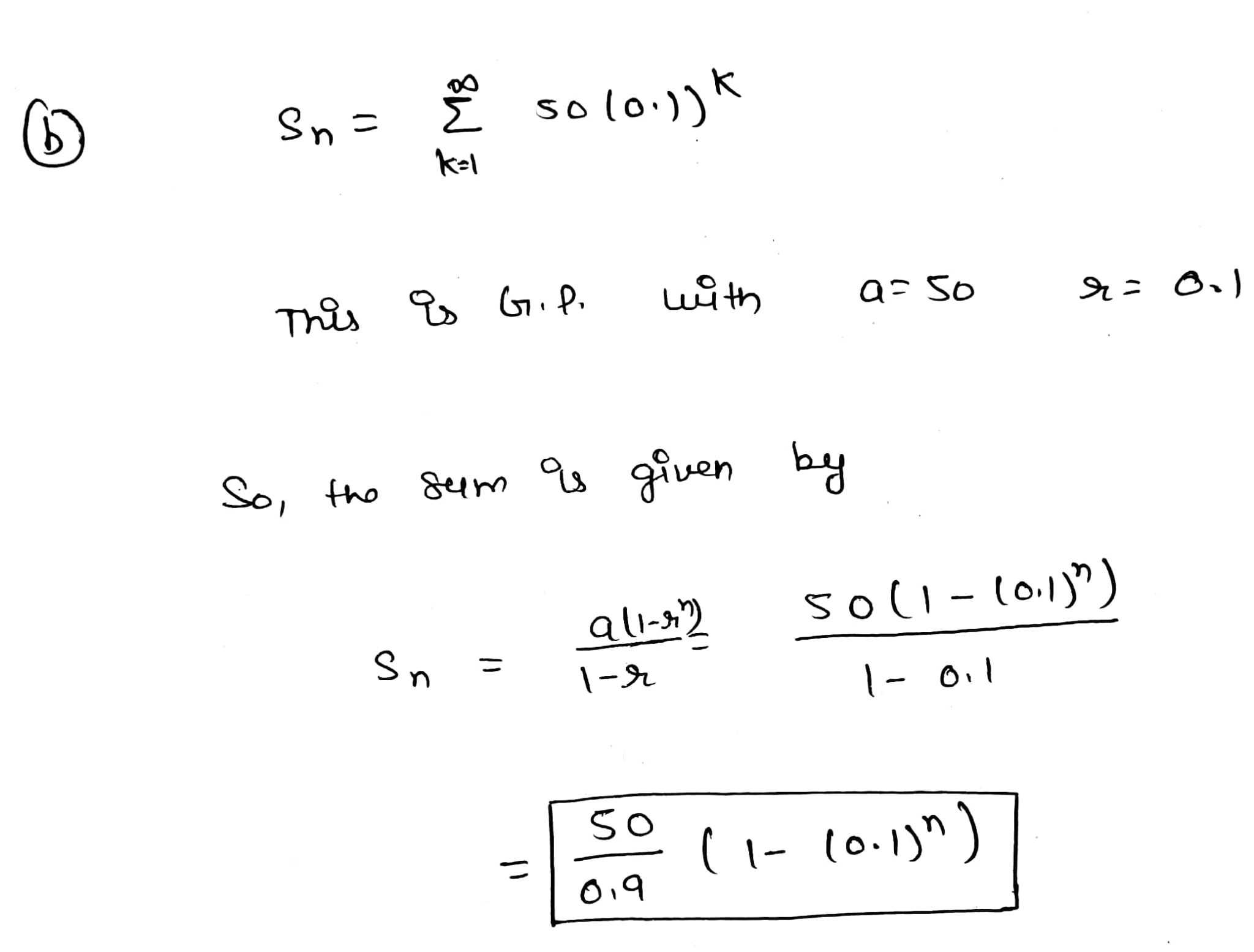#### Earn Coins

Coins can be redeemed for fabulous gifts.

Similar Homework Help Questions
• ### Consider the following infinite series. Complete parts (a) through (c) below. 00 Σ 1 4k k=1...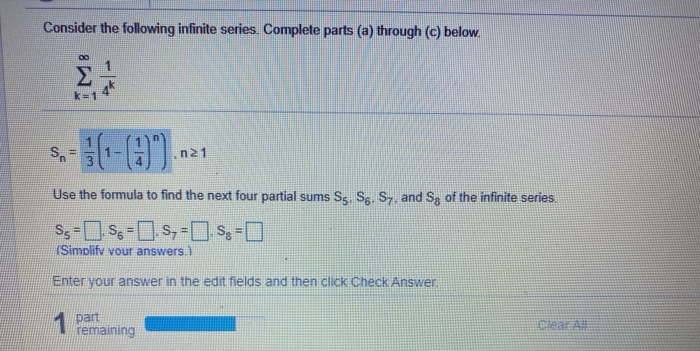Consider the following infinite series. Complete parts (a) through (c) below. 00 Σ 1 4k k=1 SA هانا (1-0). n21 Use the formula to find the next four partial sums S5 S6, S7, and Sg of the infinite series S5= 0.5-1.5, -0.5-O (Simplify vour answers.) Enter your answer in the edit fields and then click Check Answer 1 par remaining

• ### For the following telescoping series, find a formula for the nth term of the sequence of...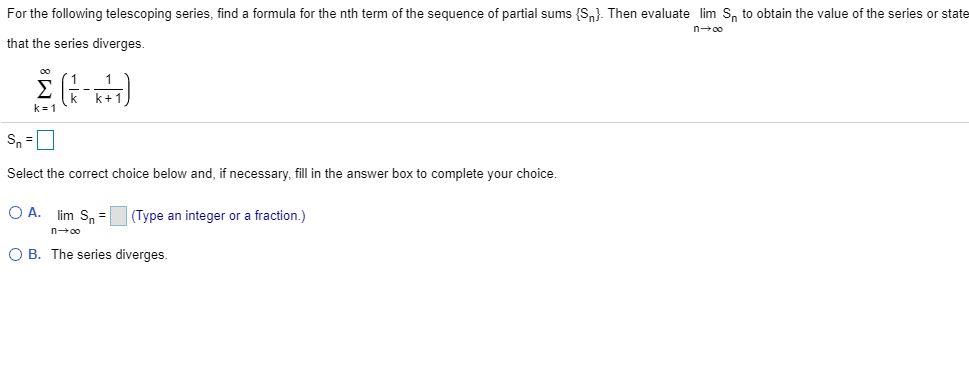For the following telescoping series, find a formula for the nth term of the sequence of partial sums {Sn}. Then evaluate lim S, to obtain the value of the series or state n-00 that the series diverges k+1 k= 1 Select the correct choice below and, if necessary, fill in the answer box to complete your choice O Alim Sn (Type an integer or a fraction.) n00 OB. The series diverges

• ### 1 3. (12,4) Let b. and consider the series (+1)*** (2n)! Answer the following: na (2n)!...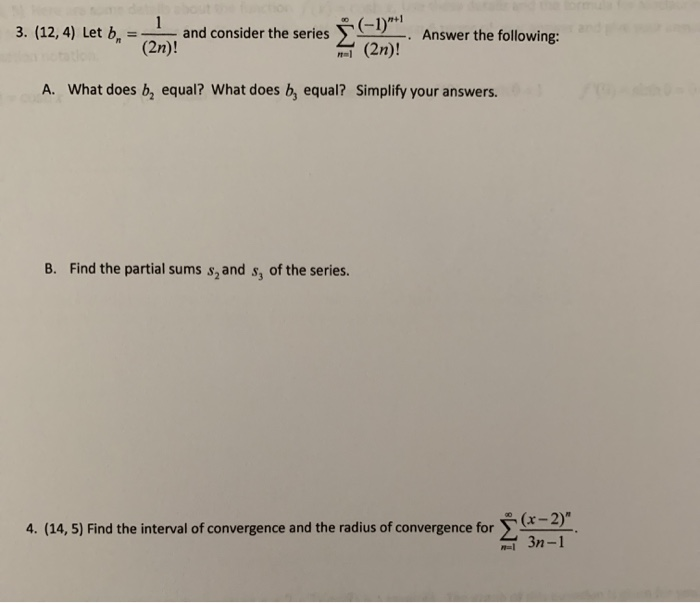1 3. (12,4) Let b. and consider the series (+1)*** (2n)! Answer the following: na (2n)! A. What does be equal? What does b, equal? Simplify your answers. B. Find the partial sums s, and s, of the series. 4. (14, 5) Find the interval of convergence and the radius of convergence for (x - 2)" 3n-1